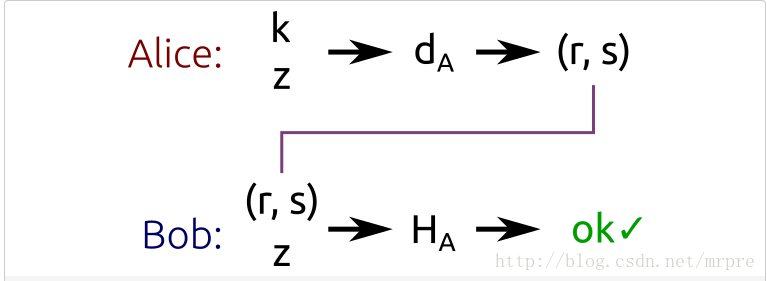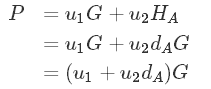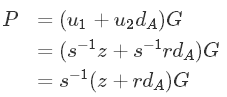# ECDSA签名算法(ECC椭圆曲线算法3)

`Alice想要用私钥签名一个数据，Bob想要使用Alice的公钥验证这个签名；只有Alice能够进行计算签名然后得到签名，每个人都能验证签名值`

ECDSA签名算法的输入是`数据的哈希值`，而不是数据的本身，至于`哈希算法`选用哪一个就取决于自己了。为了使得`ECDSA`的输入值的比特数和子群的阶n的比特数一样，哈希值可能会被截断。我们把ECDSA输入称之为`Z`

#### 算法工作流程如下：

（1）取一个范围在`[1, n - 1]`的随机数k
（2）计算点P=kG
（3）计算r = xp mod n
（4）如果 r == 0，执行第一步
（5）计算s = k^-1 (z + r*da) mod n （da是Alice的公钥，k^-1 是 k 对n的逆元）
（6）如果s==0，执行第一步
（7）二元组(r, s)就是签名值
（一般情况，最后的结果r和s是用asn1格式封装的，至少的TLS签名和数字证书签名中是这样的，不是简单的r+s这样字节直接拼接）Bob使用Alice的公钥对(r, s)和Z进行验证,我们来看看Bob如何验证的：

（1）计算u1 = s^-1 z mod n
（2）计算u2 = s^-1
r mod n
（3）计算P = u1G + u2Ha
（4）如果r == xp mod n，则验证正确

## ECDSA原理以及证明``````s = k^-1 (z + r*da) mod n
``````

da) mod n

``````kG = s^-1*(z + r *da)*G mod n = P
``````

## K的重要性

``````s1 = k^-1 (z1 + r*da) mod n等式1
s2= k^-1 (z2 + r*da) mod n等式2
``````

``````s1 -s2 = k^-1 (z1 - z2) mod n
``````

z1和z2 可以自己使用哈希算法对软件进行哈希能够获取到。s1和s2能够从前面值中获取到。所以k值就轻松的获取到了

``````K = (s1 -s2)^-1 * (z1 -z2) mod n
``````

•